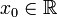# Globally analytic function

Consider a function$f$ defined on all of$\R$. We say that$f$ is globally analytic if it satisfies the following equivalent conditions:
1. There is a point$x_0 \in \R$ such that the Taylor series of$f$ at$x_0$ exists and converges to$f$ on all of$\R$.
2. There is a point$x_0 \in \R$ such that$f$ has a power series at$x_0$ that converges to$f$ on all of$\R$.
3. For every point$x_0 \in \R$, the Taylor series of$f$ at$x_0$ exists and converges to$f$ on all of$\R$.
4. For every point$x_0 \in \R$,$f$ has a power series at$x_0$ that converges to$f$ on all of$\R$.